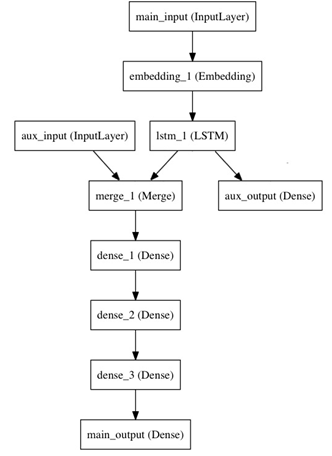zoukankan      html  css  js  c++  java
• # 函数式模型概述

Keras函数式模型接口是用户定义多输出模型、非循环有向模型或具有共享层的模型等复杂模型的途径。一句话，只要你的模型不是类似VGG一样一条路走到黑的模型，或者你的模型需要多于一个的输出，那么你总应该选择函数式模型。函数式模型是最广泛的一类模型，序贯模型（Sequential）只是它的一种特殊情况。

因为序贯模型是函数式模型的一个特例，所以我们从一个简单的序贯模型开始，举例看看函数式模型是如何完成的。

模型的主要输入是新闻本身，也就是一个词语的序列。但我们还可以拥有额外的输入，如新闻发布的日期等。

这个模型的损失函数将由两部分组成，辅助的损失函数评估仅仅基于新闻本身做出预测的情况，主损失函数评估基于新闻和额外信息的预测的情况，即使来自主损失函数的梯度发生弥散，来自辅助损失函数的信息也能够训练Embeddding和LSTM层。在模型中早点使用主要的损失函数是对于深度网络的一个良好的正则方法。总而言之，该模型框图如下：函数式模型搭建这个框图里的模型是方便的。

主要的输入接收新闻本身，即一个整数的序列（每个整数编码了一个词）。这些整数位于1到10000之间（即我们的字典有10000个词）。这个序列有100个单词。

from keras.layers import Input, Embedding, LSTM, Dense

from keras.models import Model

main_input = Input(shape=(100,), dtype='int32', name='main_input')

x = Embedding(output_dim=512, input_dim=10000, input_length=100)(main_input)

lstm_out = LSTM(32)(x)

然后，我们插入一个额外的损失，使得即使在主损失很高的情况下，LSTM和Embedding层也可以平滑的训练。

auxiliary_output = Dense(1, activation='sigmoid', name='aux_output')(lstm_out)

再然后，我们将LSTM与额外的输入数据串联起来组成输入，送入模型中：

auxiliary_input = Input(shape=(5,), name='aux_input')

x = keras.layers.concatenate([lstm_out, auxiliary_input])

x = Dense(64, activation='relu')(x)

x = Dense(64, activation='relu')(x)

x = Dense(64, activation='relu')(x)

# And finally we add the main logistic regression layer

main_output = Dense(1, activation='sigmoid', name='main_output')(x)

最后，我们定义整个2输入，2输出的模型：

model = Model(inputs=[main_input, auxiliary_input], outputs=[main_output, auxiliary_output])

模型定义完毕，下一步编译模型。我们给额外的损失赋0.2的权重。

我们可以通过关键字参数loss_weights或loss来为不同的输出设置不同的损失函数或权值。

这两个参数均可为Python的列表或字典。这里我们给loss传递单个损失函数，这个损失函数会被应用于所有输出上。

model.compile(optimizer='rmsprop', loss='binary_crossentropy', loss_weights=[1., 0.2])

编译完成后，我们通过传递训练数据和目标值训练该模型：

因为我们输入和输出是被命名过的（在定义时传递了“name”参数），我们也可以用下面的方式编译和训练模型：

model.compile(optimizer='rmsprop', loss={'main_output': 'binary_crossentropy', 'aux_output': 'binary_crossentropy'},

loss_weights={'main_output': 1., 'aux_output': 0.2})

{'main_output': labels, 'aux_output': labels},

epochs=50, batch_size=32)

• 相关阅读: